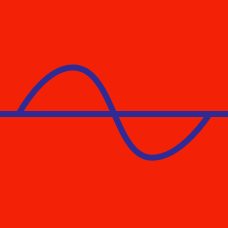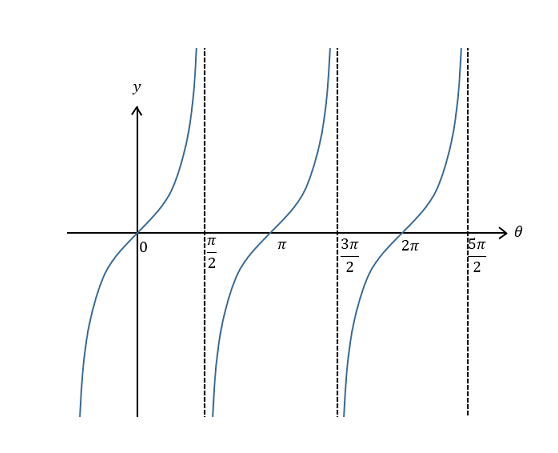Geometry

# Tangent and Cotangent Graphs

What is the value of $$\theta$$ satisfying $$8\tan^2\theta = 24$$ and $$-\frac{\pi}{2} < \theta < \frac{\pi}{2}?$$

What is the minimum value of the function $$y= \tan\theta+18,$$ where $$\frac{\pi}{3} \leq \theta < \frac{\pi}{2} ?$$

What is the range of $$\theta$$ that satisfies $$\tan\theta +6 \geq \cot\theta +6$$ and $$0 \leq \theta < \pi ?$$

What is the period of the function $$y=\cot\frac{\theta}{2} ?$$Which of the following functions corresponds to the above graph?

×